Pacific B usiness R eview (International)A Refereed Monthly International Journal of Management Indexed With Web of Science(ESCI)
ISSN: 0974-438X
Impact factor (SJIF):8.603
RNI No.:RAJENG/2016/70346
Postal Reg. No.: RJ/UD/29-136/2017-2019

## Empirical study of Impact of Financial Leverage on Dividend of Nationalized Banks in India

Dr. Manisha Goel

Associate Professor,

Department of Management Studies,

J. C. Bose University of Science and Technology, YMCA

Ruchi Mangla,

J. C. Bose University of Science and Technology, YMCA

Abstract

Dividend is one of the difficult choices that every business has to make. On one hand, dividend has the capacity to make shareholders happy to spread the positive information in the market; on the other hand it reduces the investment in projects which are capable of accelerating growth of the business and thus defeating the purpose of shareholders’ wealth maximization. Financial Leverage reflects the ability of a firm to use various fixed financial charges to magnify the effects of variation in EBIT on EPS of the firm. Since financial leverage has a capacity to increase earnings for the shareholders it must have some effect on the portion of this earning distributed to the shareholders.   The study has aimed to understand and analyze financial leverage and dividend of nationalized banks in India. The present study is a quantitative research where the relationships have been developed in the form of statistical model. A co relational research design has been adopted for the study to analyze the effect of financial leverage and dividend.

Keywords: Dividend, Financial Leverage, Earnings, Co-relation, Regression

Introduction

Dividend is very crucial financial decisions of the firm which has the capacity to affect the value of the firm. The decision affects not only the shareholders but also the financial position of the company, expansion or growth plans, liquidity, creditors, value of shares and even the perception about the company and management. Dividend as a policy decision is affected by lot of factors, which changes with country and industry concerned. Earnings, past earnings, ownership, risk, liquidity, growth opportunities, tax aspects and leverage are to name a few.  Even the magnitude and direction of impact of these factors change with change in country and industry.

Financial Leverage is being considered as a tool to multiply the returns for the shareholders by the business firms. Using the concept of financial leverage every business assumes that it will be able to generate higher returns on funds borrowed which will be passed on to the shareholder, which will increase their return. So if financial leverage has a capacity to increase earnings for the shareholders it must have some effect on the portion of this earning distributed to the shareholders. Banks are being considered as profit making business opportunity by the investors in capital markets these days. With the privatization of banks, the expectations of investors from this sector have increased.  In the present study, an attempt has been made to analyze the impact of financial leverage on the dividend paid by the nationalized banks.

Literature Review

While studying the determinants of dividend many of researches included leverage as an factor suggested that leverage could be one of the determinants. In order to analyze the impact of financial leverage on dividend paid, various studies have been explored to understand the relationship between these two variables.

Sector is relevant for dividend payment (Twaijry, 2007). Along with it country is also important. So the relationship in context to Indian banks has been explored.

Gupta and Banga (2010) researched on the various factors affecting dividend policy of a bank with the help of regression analysis. It has been made sure that the factors used during the analysis do not possess multi-co linearity problems. It also studies the effect of the financial leverage in determining the dividend policy of a firm suggesting that if the financial leverage is more than the required optimum then the firm has to bear a higher cost of transactions and the risk associated with the firm also increases. Therefore, financial leverage exhibits an inverse relationship with dividend policy especially in context of the Indian firms and it has been also found that the liquidity of the Indian firms affect the dividend decisions affirmatively.

Gupta (2012)examined the selected private and public sector banks in India and measured the effect of financial leverage on these selected banks. This study has been carried over the period from 2007 to 2011. For analyzing the results, the balance sheets of the respective banks were observed and also the effects of leverage ratio were deduced from the same. It has been observed that the financial leverage in the commercial banks did not experience a rise in consecutive years following the year 2007 rather showed a decline in this area. Whereas, the government banks in the country showed a stable leverage ratio over the years and also experienced a movement towards an increased financial leverage ratio within the banks. On the other hand, public sector banks experienced a decline in the owner funds which were measured as a percentage of total sources of public and private sector banks, whereas on the other side, the commercial banks showcased a significant increase in the funds of the owners in the bank. The fixed asset turnover ratio has been also estimated for the public and private sector banks from 2007 to 2011 and it has been found that fixed assets turnover ratio increased in both the sectors of the banks but with the public sector experiencing a higher turn-over of the fixed assets compared to that experienced by the commercial banks in the country.

Another research (Sri Hari et al., 2012) concluded that nationalized sector banks paid more dividends than private sector banks.

Dr. Souvik Banerjee and Dr. K.T. Rangamani analysed the dividend paid by 40 banks in India for the period of 2010-2015. They took 24 public sector and 16 private sector banks to conduct their study. They concluded that dividend payout ratio of private banks and public banks were not statistically different from each other.

Research Design

The present research is quantitative research conducted to analyze the properties of the past records and to make predictions for the future by developing mathematical models. In social sciences like commerce, management and economics statistical methods are extensively used in quantitative research.  In the present research, the quantitative values for dividend, financial leverage have been studied for a period of 13 years. The values for the years where banks have paid no dividend have been ignored. Inferences have been drawn from the values collected for 19 nationalized banks. Further the relationships have been developed in the form of statistical models. The focus of the study has been to explore the impact of financial leverage on dividend paid by the banks. Correlation and regression models have been developed for the leverage, earnings and dividend, of nationalized banks in India. For the purpose of analysis, SPSS 21.0 has been used to explore correlation and regression.

Data Analysis and Interpretation:

The regression has been used as a tool to find out the impact of financial leverage on DPS of nationalized banks in India with DPS as dependent variable and financial leverage as independent variable.

Linear Regression of DPS and Fl for Nationalized Banks

The linear regression has been run on the data related to nationalized banks to find out the impact of their financial leverage on dividend per share (DPS). The results have been depicted as below;

Table 1 Regression of  DPS and FL of  Nationalized Banks

 DPS Financial Leverage Pearson Correlation DPS 1.000 -.396 Financial Leverage -.396 1.000 Sig. (1-tailed) DPS . .000 Financial Leverage .000 .

Table 2   Model Summary of Regression of  DPS and FL of Nationalized Banks

 R R Square Adjusted R Square Std. Error of the Estimate Durbin-Watson .396b .157 .152 4.79607 .512

Table 3 Coefficients of  DPS and FL of  Nationalized Banks

 Unstandardized Coefficients Standardized Coefficients t Sig. B Std. Error Beta (Constant) 13.218 1.338 9.880 .000 Financial Leverage -.403 .069 -.396 -5.794 .000

From the above tables it has been concluded that there is significant negative relationship between DPS and financial leverage of nationalized banks as the p value is less than 0.05. DPS has decreased with the increase in financial leverage in case of nationalized banks in India. The regression coefficient is -0.403 with a constant of 13.218.

REGRESSION MODEL

The equation can be written as a model fit equation between two variables as-

DPS = 13.218 – 0.403(Financial Leverage)

As the value ofR2  is .157 , it means that only 15.7 % of variations in  DPS are explained by Financial Leverage for   the  nationalized banks. The null hypothesis that there is no significant impact of financial leverage on equity dividend of nationalized banks has been rejected since the p value is less than 0.05. It shows that there is significant impact of financial leverage on equity dividend paid by nationalized banks.

Validity of Regression Results for DPS and Fl of Nationalized Banks

Before we reach any conclusion regarding this model it is necessary to ensure the validity of regression results. To check validity of results of regression few basic assumptions of classic linear regression model are checked with -. tests of linearity, normality, stationarity, auto collinearity and homoscedasticity.

1. Assumption of Linearity

This has been checked with the help of Scatter diagram. The scatter diagram of the above said data is represented in figure below;

Figure 1 Scatter Plot of DPS and  FL for Nationalized banks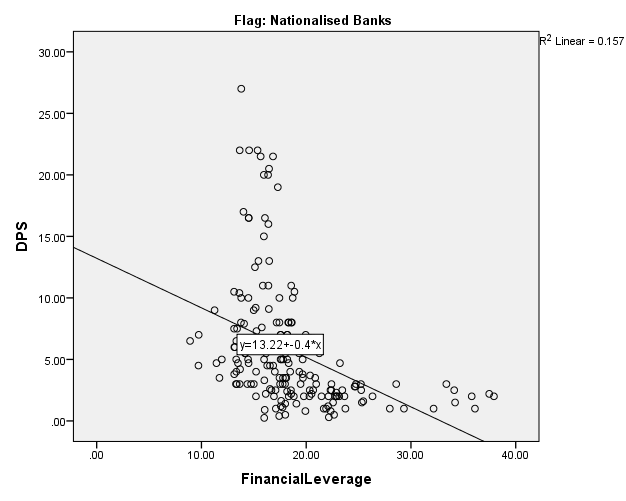As there are number of outliers present and a cluster is formed, it has been observed that the relationship is not linear between Financial Leverage and DPS of nationalized banks.

1. Assumption of Normality

Shapiro-Wilk test along with Q-Q plot has been used to test the normality of data.

1. Shapiro-Wilk Test- the results of the test are as below:-
2.

Table 4 Test of Normality of DPS and FL of  Nationalized Banks

 Kolmogorov-Smirnovb Shapiro-Wilk Statistic df Sig. Statistic Df Sig. DPS .172 182 .000 .795 182 .000 Financial Leverage .144 182 .000 .881 182 .000

As we are able to see that level of Significance for Shapiro-Wilk test is below 0.05, so the data don’t hold the assumption of normality and regression results of the data which is not normal, are not valid.

1. Q-Q Plot –On the observation of the data of DPS on Q-Q plots, it is found that it is quite along the expected line but this is not the case of Log Financial Leverage.

Figure 2  Q-Q plot of   DPS of  Nationalized Banks      Figure 3 Q-Q plot of  FL of Nationalized  Banks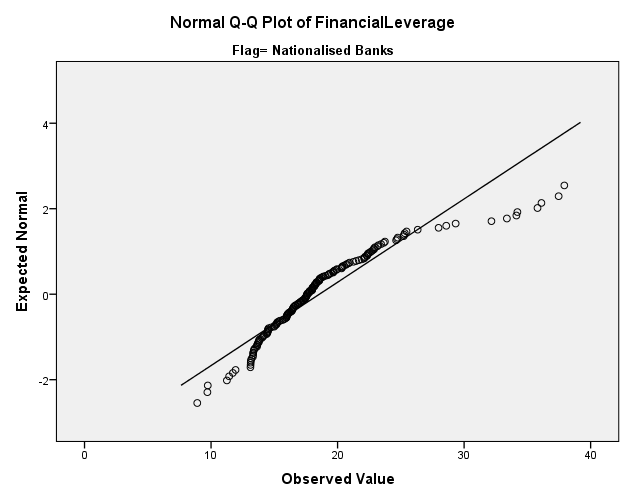1. Assumption of Stationarity  and auto correlation

Stationarity has been checked using auto correlation test in SPSS and Durbin-Watson statistics.

1. Auto-correlation  test  Auto correlation test results show that data is not stationary

Figure 4 ACF Chart of  FL of NationalizedFigure 5 ACF Chart of  DPS of Nationalized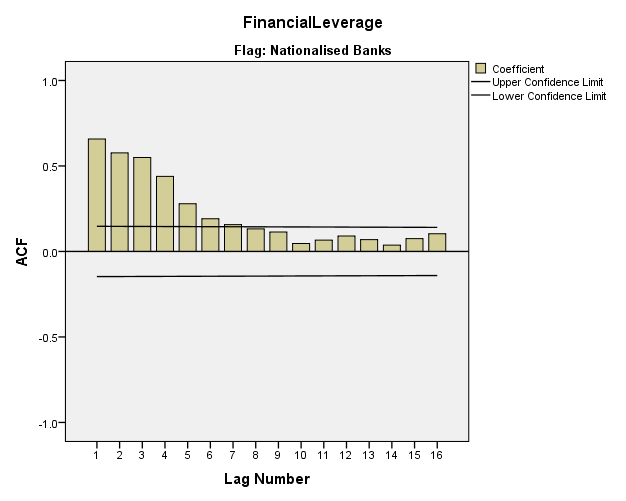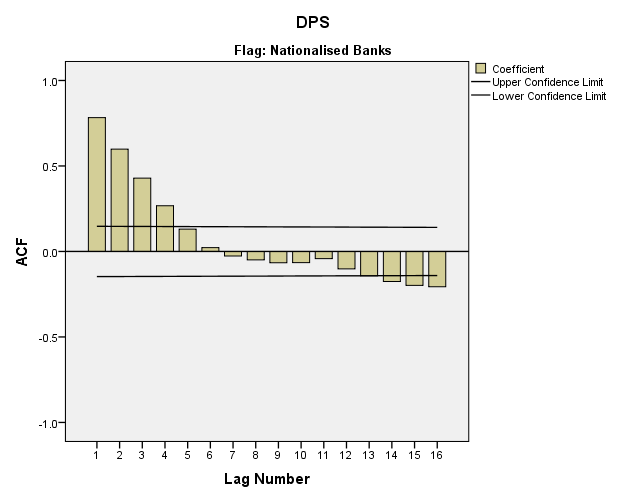1. Durbin –Watson  Statistics

As per the model summary, the Durbin-Watson value is 0.512 which very far off from the expected value of 2, for that to fulfill the assumption. So the data is having auto- colinearity.

1. Assumption of Homoscedasticity

To check this assumption the scatter plot of residuals has been observed.

Figure 6 Scatter Plot of Regression Standardized Residuals of FL and DPS of Nationalized Banks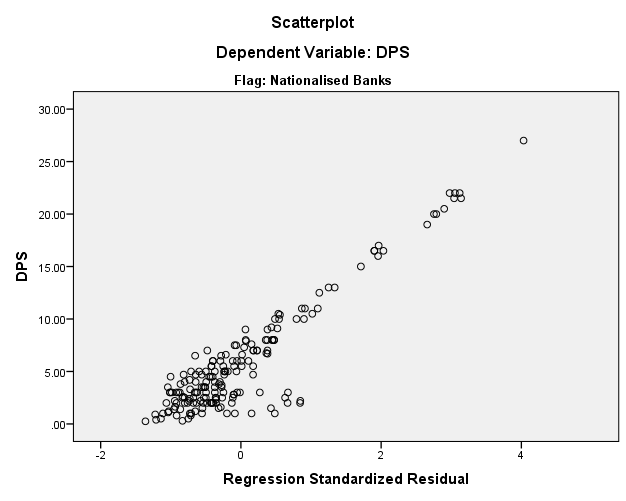The scatter plot of residuals is not equally distributed and depicts a cluster, which suggests the presence of hetroscedasticity

1. Assumption of Correct Regression

This assumption is checked with the help of P-P plot of observed and expected residuals. As per the observed P-P plot the residuals are near to but not exactly on the expected line. So the egression is not a good fit.

Figure 7   Normal P-P Plot of Regression Standardized Residuals of  FL and   DPS of Nationalized Banks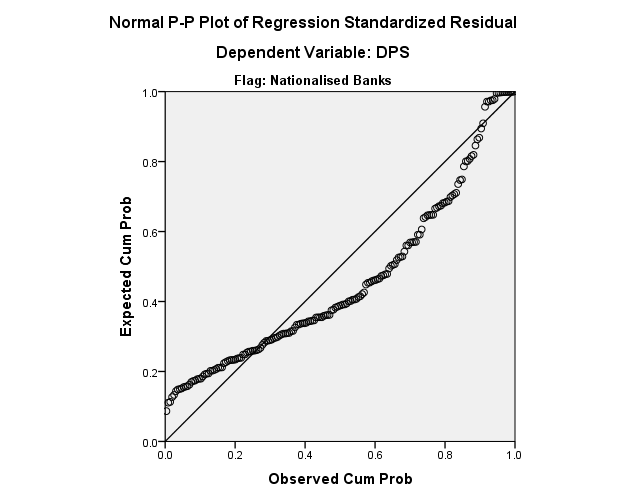It has been analyzed that the majority of assumption of linear regression model are not satisfied in the case of nationalized banks also, so some kind of transformation is needed to make the data normal and fit the regression line. This transformation has been done by taking log values.

Linear Regression with Log of DPS and Fl for Nationalized Banks

The transformation has been done with the help of taking log of all the values. After transformation the model has been developed in the form of:-

Log DPS = a + b (Log Financial Leverage)

The regression has been run on the data related to nationalized banks and the details are as follows:-

Table 5 Linear Regression with Log of DPS and FL of Nationalized Banks in India

 Correlationsa Log DPS Log Financial Leverage Pearson Correlation Log DPS 1.000 -.488 Log Financial Leverage -.488 1.000 Sig. (1-tailed) Log DPS . .000 Log Financial Leverage .000 .

Table 6 Model Summary of Linear Regression with Log of DPS and FL of Nationalized Banks

 R R Square Adjusted R Square Std. Error of the Estimate Durbin-Watson .488 .238 .233 .33855 .808 Table 7 Coefficients of Linear Regression with Log of  DPS and FL of  Nationalized Banks Unstandardized Coefficients Standardized Coefficients t Sig. B Std. Error Beta (Constant) 2.763 .290 9.539 .000 Log Financial Leverage -1.723 .230 -.488 -7.492 .000

From the above tables it has been observed that there exists significant negative relationship between Log DPS and Log Financial Leverage of nationalized banks as the p value is less than 0.05.  Log Financial Leverage has a negative impact on Log DPS. It means with the increase in leverage, DPS decreases in case of nationalized banks in India. The regression coefficient is -1.723 with a constant of 2.763.

Regression Model

The equation can be written as a model fit equation between two variables as-

Log DPS = 2.763 – 1.723(Log Financial Leverage)

As the value ofR2  is .238 , it means that  23.8 % of variations in  DPS are explained by Financial Leverage for  the  nationalized banks.

Validity of Regression Results with Log DPS and Log Fl for Nationalized Banks

Before we reach any conclusion regarding this model it is necessary to ensure the validity of results of regression. To check validity of results of regression few basic assumptions of classic linear regression model are checked with - tests of linearity, normality, stationarity, auto collinearity and homoscedasticity.

1. Assumption of Linearity

This has been checked with the help of Scatter diagram. The scatter diagram of the above said data has been represented in figure 8.

Figure 8 Scatter Plot of Log DPS and Log FL for Nationalized Banks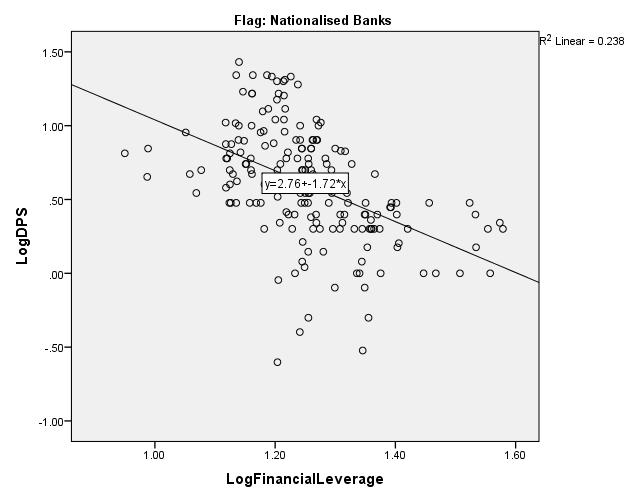.

As there are number of outliers present and a cluster is formed, it has been observed that the relationship is not linear between Log Financial Leverage and Log DPS of nationalized banks.

1. Assumption of Normality

Shapiro-Wilk test along with Q-Q plot has been used to test the normality of data.

1. Shapiro-Wilk Test- the results of the test are as below:-
2.

Table 8  Test of Normality of  Log DPS and   Log FL of NationalizedBanks

 Kolmogorov-Smirnova Shapiro-Wilk Statistic df Sig. Statistic Df Sig. Log DPS .076 182 .012 .986 182 .070 Log Financial Leverage .091 182 .001 .968 182 .000

As we are able to see that level of Significance for Shapiro-Wilk test is below 0.05 for Log financial leverage, so the data don’t hold the assumption of normality and regression results of the data which is not normal, are not valid.

1. Q-Q Plot – On the observation of the data on Q-Q plots, it is found that it is not normal.

Figure 9  Q-Q plot of   Log DPS of Nationalized Banks           Figure 10 Q-Q plot of Log FL of Nationalized Banks

1. Assumption of Stationarity  and auto correlation

Stationarity has been checked using auto correlation test in SPSS and Durbin-Watson statistics.

1. Auto-correlation  test  Auto correlation test results show that data is not stationary

Figure 11  ACF Chart of Log FL of Nationalized      Figure 12  ACF Chart of Log DPS of Nationalized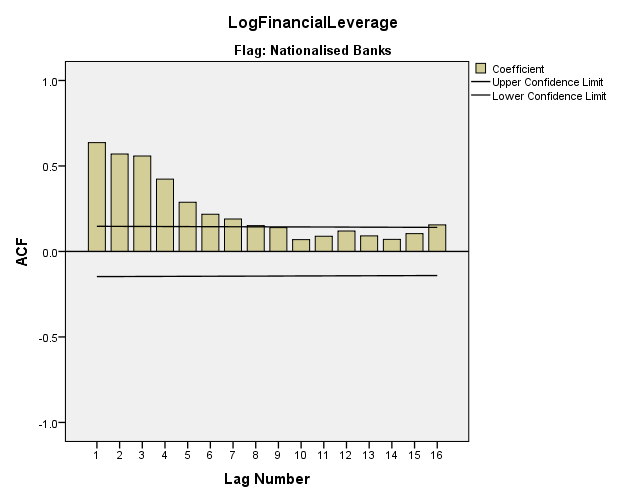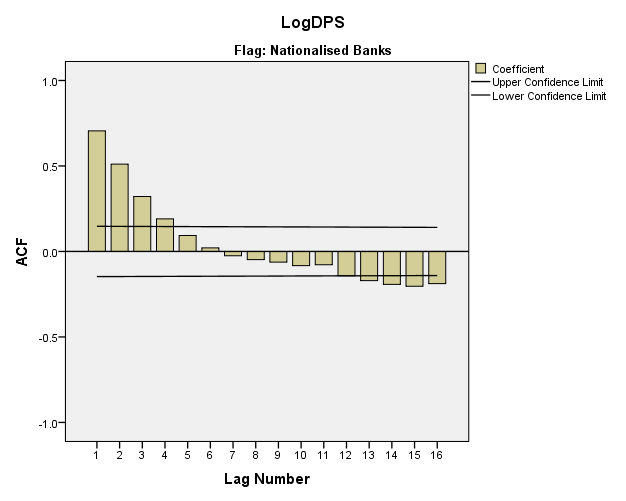1. Durbin –Watson  Statistics

As per the model summary, the Durbin-Watson value is 0.808 which very far off from the expected value of 2, for that to fulfil the assumption. So the data is having auto- colinearity.

1. Assumption of Homoscedasticity

To check this assumption the scatter plot of residuals has been observed.

Figure 13 Scatter Plot of Regression Standardized Residuals of

Log FL and Log DPS of Nationalized Banks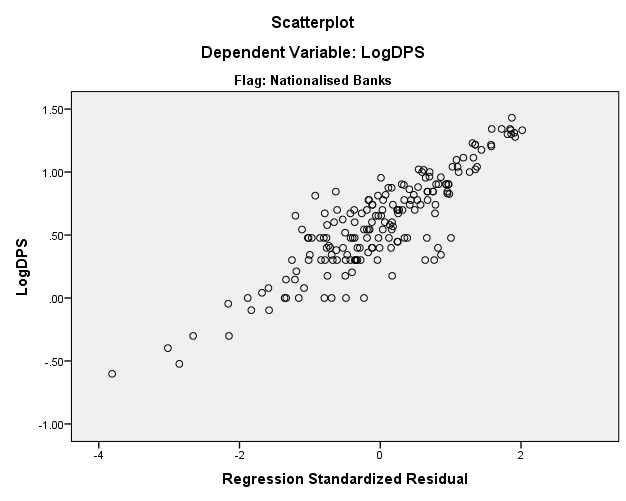The scatter plot of residuals is not equally distributed, which suggest the presence of hetroscedasticity.

1. Assumption of Correct Regression

This assumption is checked with the help of P-P plot of observed and expected residuals.

Figure14  Normal P-P Plot of Regression Standardized Residuals of

Log FL and  Log DPS of Nationalized banks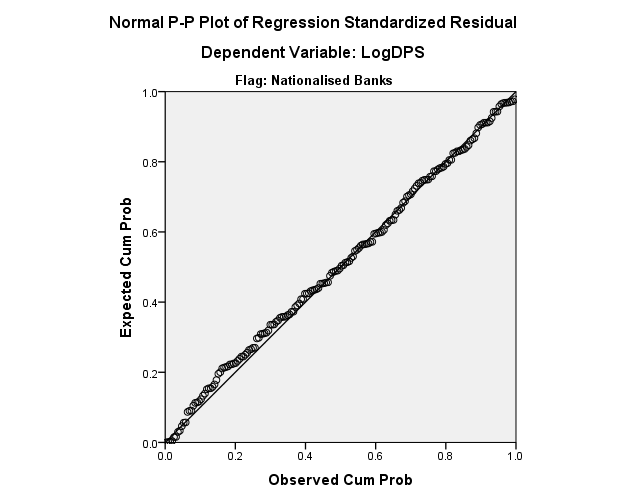As per the observed P-P plot the residuals are very much lying on the expected line. So this assumption is fulfilled.

It has been analyzed that in this case also all of assumption of linear regression model are not satisfied. Though the results have improved as compared to simple regression but regression line cannot be taken as a good fit. Now the efforts have been made to find the non linear regression between the variables.

Nonlinear Regression of DPS and Fl of Nationalized Banks

As the linear regression model even after transformation with log is not a good fit to explain the impact of financial leverage on dividend of nationalized banks, Non-linear regression model has also been applied. The correlation between dividend paid and financial leverage is negative, therefore decay model has been chosen.

The model is in the form as below:-

DPS= A-(B*(C*Financial Leverage))

When the same model  has been   run on the data related to nationalized banks, the results are as below:-

Table 10 Parameter Estimate for Non-Linear Regression of DPS and FL for Nationalized Banks

 Parameter Estimate Std. Error 95% Confidence Interval Lower Bound Upper Bound A 13.218 1.342 10.570 15.865 B -.748 5632898.382 -11115429.734 11115428.237 C -.538 4047563.080 -7987078.757 7987077.682

From the above tables it has been concluded that there is a negative relationship between DPS and Financial Leverage nationalized of banks i.e. with the increase in leverage DPS decreases in case of   nationalized banks.

Nonlinear Regression Model

The equation can be written as a model fit equation between two variables as-

DPS= 13.218 - (-0.748*(-0.538*Financial Leverage)) i.e.

DPS= 13.218 - (0.748*(0.538*Financial Leverage))

As the value ofR2  is .157 , it means that  15.7 % of variations in  DPS are explained by Financial Leverage for  the  nationalized banks.

Conclusion:

As per the results of the analysis there exist a negative relationship between financial leverage and dividend paid by nationalized banks in India. Linear regression models after transformation with Log   have also been developed depicting the significant impact of FL on DPS for banks. Though the models cannot be used to predict DPS on the basis of FL yet the results of the study can be used by the financial experts to formulate strategies related to capital structure.

References

Aasia Asif, WaqasRasool, and Yasir Kamal, "Impact of financial leverage on dividend policy: Empirical evidence from Karachi Stock Exchange-listed companies." African Journal of Business Management, vol. 5, no.4, 2011, pp. 1312.

C. Bryan Cloyd, John Robinson, and Connie Weaver, "Does Ownership Structure Affect Corporations' Responses to Lower Dividend Tax Rates? An Analysis of Public and Private Banks." 2005.

Dinesh Kumar Sharma and RituWadhwa, "Determinants of Dividend Policy Decision: An Analysis of Banks in India." Proceedings of International Conference on Strategies in Volatile and Uncertain Environment for Emerging Markets, 2017.

Duha Al-Kuwari, "Determinants of the Dividend Policy of Companies Listed on Emerging Stock Exchanges: The Case of the Gulf Cooperation Council (GCC) Countries." 2009.

Eugene F Fama and Harvey Babiak, "Dividend policy: An empirical analysis." Journal of the American statistical Association, Vol. 63, no.324, 1968, pp.1132-1161.

H. Kent Baker and Gary E. Powell, "Determinants of corporate dividend policy: a survey of NYSE firms." Financial Practice and education, vol.10, 2000, pp. 29-40.

H. Kent Baker, E. Theodore Veit, and Gary E. Powell. "Factors influencing dividend policy decisions of Nasdaq firms." Financial Review, vol. 36, no.3, 2001, pp.19-38.

HashimZameer, ShahidRasool, Sajid Iqbal, and UmairArshad."Determinants of dividend policy: A case of banking sector in Pakistan." Middle-East Journal of Scientific Research, Vol.18, no. 3, 2013, pp. 410-424.

Husam-Aldin Nizar Al-Malkawi, "Factors influencing corporate dividend decision: Evidence from Jordanian panel data." International Journal of Business, vol.13, no.2, 2008, pp.177.

Jorge Farinha, "Dividend policy, corporate governance and the managerial entrenchment hypothesis: an empirical analysis." Journal of Business Finance & Accounting, Vol.30, no.910, 2003, pp.1173-1209.

Lee Wei Sim, "A study on leading determinants of dividend policy in Malaysia listed companies for food industry under consumer product sector." Conference Master Resources, 2011.

M. Sudhahar and T. Saroja, "Determinants of dividend policy in Indian banks: An empirical analysis." IUP Journal of Bank Management, Vol.9, no.3, 2010, pp. 63.

M. Z Alam and Mohammad Emdad Hossain, "Dividend policy: a comparative study of UK and Bangladesh based companies." IOSR Journal of Business and Management, vol.1,no.1, 2012, pp.57-67.

ManojAnand, "Factors influencing dividend policy decisions of corporate India." 2004.

Mohammad Tamimi and NasrollahTakhtaei, "Relationship between Firm Age and Financial Leverage with Dividend Policy." Asian Journal of Finance & Accounting, Vol. 6, no.2, 2014, pp. 53.

Mokhtar Emamalizadeh, Mousa Ahmadi, and JaffarPouyamanesh, "Impact of financial leverage on dividend policy at Tehran Stock Exchange: A case study of food industry." African Journal of Business Management, Vol.7, no.34, 2013, pp. 3287.

Ross N Dickens, K. Michael Casey, and Joseph A. Newman, "Bank dividend policy: explanatory factors." Quarterly journal of Business and Economics,2002, pp. 3-12.

S. JasvirSura, Karam Pal, and B. S. Bodla, "Factors Influencing Dividend Policy Decisions in Banking Sector: An Indian Evidence." 2012.

Souvik Banerjee and K. T. Rangamani, "A Comparative Analysis of Dividend Policy of Public and Private Sector Banks in India." SAMVAD, vol. 11, 2016, pp. 59-64.

Turki SF Alzomaia, and Ahmed Al-Khadhiri, "Determination of dividend policy: The evidence from Saudi Arabia." International Journal of Business and Social Science, vol. 4, no.1, 2013.